Next: About this document ... Up: lab_template Previous: lab_template

Subsections

# Contour lines, directional derivatives, and the gradient

## Getting Started

To assist you, there is a worksheet associated with this lab that contains examples. You can copy the worksheet to your home directory with the following command, which must be run in a terminal window, not Maple. Go to the Start menu on the computer. In the bottom search rectangle type putty and open the putty terminal. Log in to putty as you usually log in. then copy and paste the following command:

cp /math/calclab/MA1024/Pardiff_grad_start.mws My_Documents


Now it is in your file. Open Maple and open the worksheet. Read the lab on the internet then go through the worksheet, hitting enter to see what the commands do.

## Background

### Contour lines

For functions of two variables in Cartesian coordinates, the graph is a set of points
in three-dimensional space. For this reason, visualizing functions of two variables is usually more difficult. The idea is that a planeintersects the surface in a curve. The projection of this curve on the
plane is called a level curve. A collection of such curves for different values of
is a representation of the surface called a contour plot.

### Directional derivatives

The partial derivatives
andof
can be thought of as the rate of change ofin the direction parallel to theand
axes, respectively. The directional derivative
, where
is a unit vector, is the rate of change ofin the direction. There are several different ways that the directional derivative can be computed. The method most often used for hand calculation relies on the gradient, which will be described below. It is also possible to simply use the definition
to compute the directional derivative. However, the following computation, based on the definition, is often simpler to use.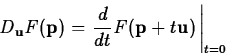One way to think about this that can be helpful in understanding directional derivatives is to realize that
is a straight line in the
plane. The plane perpendicular to theplane that contains this straight line intersects the surfacein a curve whose
coordinate is. The derivative ofat
is the rate of change ofat the point
moving in the direction.

### The Gradient

The gradient of, written
, is most easily computed as
As described in the text, the gradient has several important properties, including the following.
• The gradient can be used to compute the directional derivative as follows.
• The gradient
points in the direction of maximum increase of the value ofat.
• The gradientis perpendicular to the level curve ofthat passes through the point.

## Exercises

1. Consider the following function.
First, plot the graph of this function over the domainand
using the plot3d command. Then use the contourplot command to generate a contour plot ofover the same domain having 22 contour lines.

2. Consider again the functionfrom the first exercise. Using either method from the Getting Started worksheet, compute the directional derivative ofat the point
,in the three directions below.
A)
B)
C)
3. Using the method from the Getting Started worksheet, plot the gradient field and the contours ofon the same plot. Use the domain of
and
. Use 20 contours and a 20 by 20 gradient field. Can you use this plot to explain the values for the directional derivatives you obtained in the previous exercises? By explaining the values, I only mean can you explain what kind of surface it is and why the values were positive, negative, or zero in terms of the contours and the gradient field?Next: About this document ... Up: lab_template Previous: lab_template
Jane E Bouchard
2010-09-20